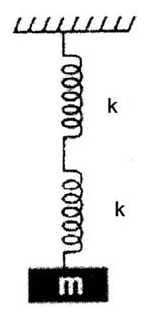# OSCILLATIONS AND WAVES

• Home -
• OSCILLATIONS AND WAVES

OSCILLATIONS AND WAVES - Topic Wise Multiple Choice Questions (MCQ)

Topic Wise free Oscillations and waves MCQ with solutions for 11th 12th exam Practice Multiple Choice Questions and answer for JEE-mains State CET Engineering Entrance GATE free Online Test

1 ) Free Physics Questions Online Mock Test Series By Cracktest

If the equation for a particle performing S.H.M. is given by y = sin 2t + √3 cos 2t, its periodic time will be …….. s.

21
π

View Solution

2 )

A person standing in a stationary lift measures the periodic time of a simple pendulum inside the lift to be equal to T. Now, if the lift moves along the vertically upward direction with an acceleration of (g/3), then the periodic time of the lift will now be……………

√3T
√(3/2)T
(T/3)
(T / √3)

View Solution

3 )

If the equation for displacement of two particles executing S.H.M. is given by y1 = 2 sin (10t + θ) and y2 = 3 cos 10t respectively, then the phase difference between the velocity of two particles will be ……….

θ
θ – (π/2)
– θ
θ + (π/2)

View Solution

4 )

When a body having mass m is suspended from the free end of two springs suspended from a rigid support, as shown in figure, its periodic time of oscillation is T. If only one of the two springs are used, then the periodic time would be ………(T / √2)
(T/2)
√2 T
2T

View Solution

5 )

If the maximum velocity of two springs (both has same mass) executing S.H.M. and having force constants k1 and k2 respectively are same, then the ratio of their amplitudes will be ……..

Select correct option from following options

(A) (k1 / k2)                    (B) (k2 / k1)

(C) √(k1 / k2)                   (D) √(k2 / k1)

A
B
C
D

View Solution